## Area in Square Centimetres (cm2) and Square Metres (m2)

Practice Unlimited Questions

#### What are square metres (meters) and square centimetres (centimeters)?

Area is measured in square centimetre (cm2) and square metre (m2).
A square of sides 1 cm (shown below) has an area of 1 square centimetre, also written as 1 cm2.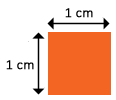A square of sides 1 m (shown below) has an area of 1 square metre, also written as 1 m2.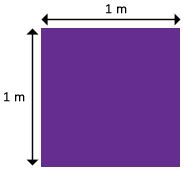#### 1. What is the area of the shaded figure below?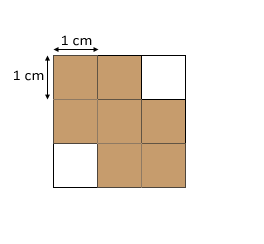The figure is made up of seven 1-cm squares.
The area of each 1-cm square is 1 cm2.
So, the area of the shaded figure is 7 cm2.

#### 2. Mr. Alex arranges a few 1-m2 marble tiles on a floor as shown below. What area of the floor is covered by the marble tiles?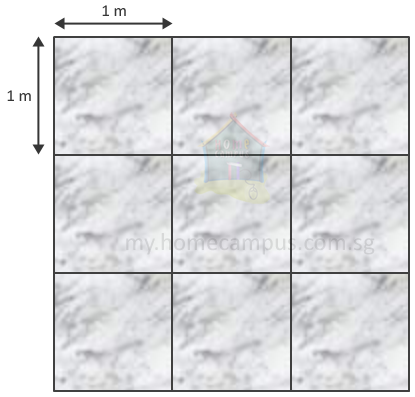Count the number of 1-m squares.
There are nine 1-m square marble tiles used in the pattern.
The area of each 1-m square is 1 m2.
So, the area of the floor covered by the marble tiles is 9 m2.
The tiles are arranged into a 3 m  ×  3 m square.

#### 3. Find the area of the figures below.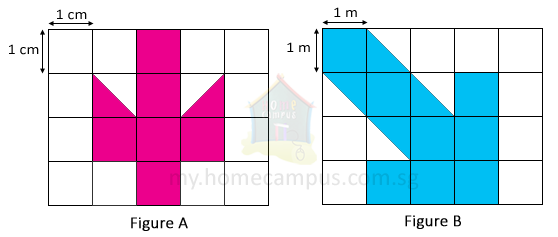Figure A:
The figure is made up of squares and half-squares.
Total area of Figure A
=  6 cm2 + 1 cm2
=  7 cm2
The area of Figure A is 7 cm2.

Figure B:
The figure is made up of squares and half-squares.
Total area of Figure B
=  8 m2 + 2 m2
=  10 m2
The area of Figure B is 10 m2.
Two half 1-cm squares make one full 1-cm square.